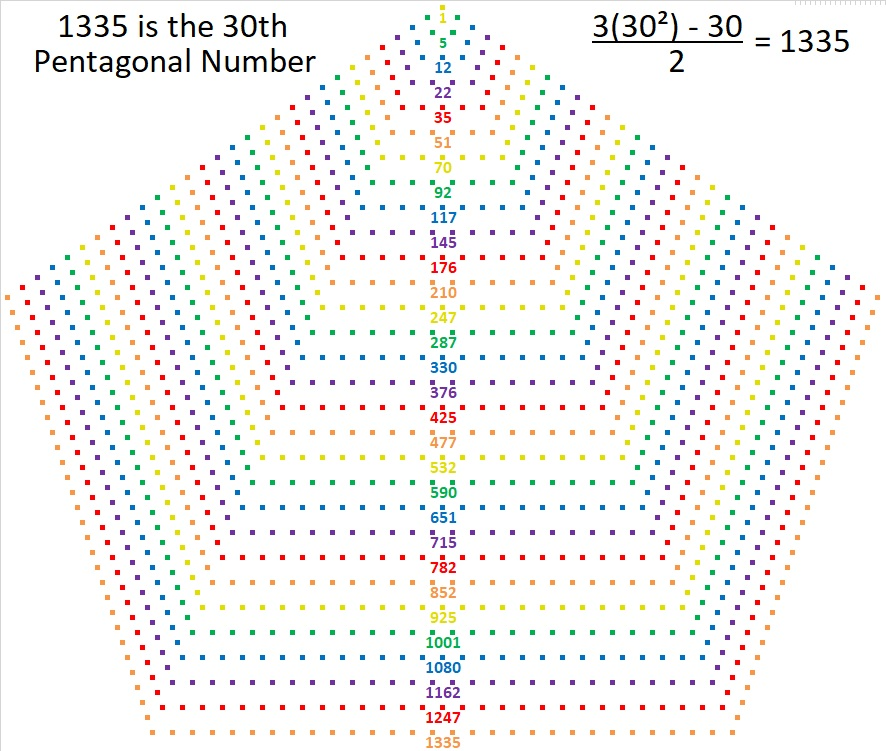1335 is a Pentagonal Number

1335 is the 30th Pentagonal Number. Here’s a graphic to help you visualize that fact:Here are some more facts about the number 1335:

• 1335 is a composite number.
• Prime factorization: 1335 = 3 × 5 × 89
• 1335 has no exponents greater than 1 in its prime factorization, so √1335 cannot be simplified.
• The exponents in the prime factorization are 1, 1, and 1. Adding one to each exponent and multiplying we get (1 + 1)(1 + 1)(1 + 1) = 2 × 2 × 2 = 8. Therefore 1335 has exactly 8 factors.
• The factors of 1335 are outlined with their factor pairs in the graphic below.1335 is also the hypotenuse of FOUR Pythagorean triples:
252-1311-1335 which is 3 times (84-437-445)
585-1200-1335 which is 15 times (39-80-89)
609-1188-1335 which is 3 times (203-396-445)
801-1068-1335 which is (3-4-5) times 267

This site uses Akismet to reduce spam. Learn how your comment data is processed.